• # 数理逻辑基础

## 第一章 命题演算

命题演算是最简单、最基本的形式系统，主要用来表示较为简单的逻辑推理的一种数学模型. 其简单性表现为：当它把复合命题分解成简单命题后，就把简单命题视为最小考察对象，不再继续对简单命题进行分解.

### 1.1 命题与命题联结词

#### 1.1.1 命题

定义1.1 命题

具有确切真值的陈述句（或断言）称为命题（proposition）.

命题的取值称为真值. 真值只有“真”和“假”两种，分别用 $\mathrm{T}$$\mathrm{T}$$1$$1$）和 $\mathrm{F}$$\mathrm{F}$$0$$0$）表示.

命题的真值非真即假，只有两种取值，这样的系统称为二值逻辑系统.

注意：

• 命题一定是通过陈述句来表达；反之，并非一切的陈述句都是命题.

• 命题的真值有时可明确给出，有时还需要依靠环境条件、实际情况、时间等才能确定其真值，但其真值一定是唯一确定的.

命题的类型：

• 若一个命题已不能分解成更简单的命题，则该命题叫原子命题或本原命题或简单命题（simple proposition）；

• 若一个命题可以分解为简单命题，而且这些简单命题之间是通过关联词和标点符号复合而构成的，则该命题叫复合命题（compound proposition）.

命题通常用大写英文字母（可带下标）来表示.

#### 1.1.2 命题联结词

命题通常可以通过一些联结词复合而构成新的命题，这些联结词被称为逻辑联结词. 在命题演算中，这些联结词可以看作运算符，因此也叫作逻辑运算符.

定义1.2 否定联结词

$P$ 是任一命题，复合命题”非 $P$ “（或”$P$ 的否定“）称为 $P$ 的否定式（negation），记作 $\mathrm{¬}P$$\lnot P$，”$\mathrm{¬}$$\lnot$“ 称为否定联结词.

$\mathrm{¬}P$$\lnot P$ 为真当且仅当 $P$ 为假.

定义1.3 合取联结词

$P$$Q$$Q$ 是任意两个命题，复合命题 ”$P$ 并且 $Q$$Q$ “（或“ $P$$Q$$Q$ ”）称为 $P$$Q$$Q$ 的合取式（conjunction），记作 $P\wedge Q$$P\land Q$，“$\wedge$$\land$” 称为合取联结词.

$P\wedge Q$$P\land Q$ 为真当且仅当 $P$$Q$$Q$ 同为真；$P\wedge Q$$P\land Q$ 为假当且仅当 $P$$Q$$Q$ 二者至少有一为假.

定义1.4 析取联结词

$P$$Q$$Q$ 是任意两个命题，复合命题“ $P$$Q$$Q$ ” 称为 $P$$Q$$Q$ 的析取式（disjunction），记作 $P\vee Q$$P\lor Q$，“$\vee$$\lor$” 称为析取联结词.

$P\vee Q$$P\lor Q$ 为真当且仅当 $P$$Q$$Q$ 至少一个为真.

这里的“或”是“相容或”（“可兼或”）.

定义1.5 蕴涵联结词

$P$$Q$$Q$ 是任意两个命题，复合命题“如果 $P$ 那么 $Q$$Q$ ” 称为 $P$$Q$$Q$ 的蕴涵式（implication），记作 $P\to Q$$P\to Q$，“$\to$$\to$” 称为蕴涵联结词，$P$ 称为蕴涵式的前提、假设或前件，$Q$$Q$ 称为结论或后件.

$P\to Q$$P\to Q$ 为真当且仅当 $P$ 为真 $Q$$Q$ 为假.

蕴涵式 $P\to Q$$P\to Q$ 可以用多种方式陈述：

• “若 $P$，则 $Q$$Q$ ”；

• $P$$Q$$Q$ 的充分条件”；

• $Q$$Q$$P$ 的必要条件”；

• $Q$$Q$ 每当 $P$ ”；

• $P$ 仅当 $Q$$Q$ ”.

给定命题 $P\to Q$$P\to Q$

• $Q\to P$$Q\to P$ 是其逆命题；

• $\mathrm{¬}P\to \mathrm{¬}Q$$\lnot P\to\lnot Q$ 是其反命题；

• $\mathrm{¬}Q\to \mathrm{¬}P$$\lnot Q\to\lnot P$ 是其逆反命题.

定义1.6 等价联结词

$P$$Q$$Q$ 是任意两个命题，复合命题“ $P$ 当且仅当 $Q$$Q$ ” 称为 $P$$Q$$Q$ 的等价式（equivalence），记作 $P↔Q$$P\leftrightarrow Q$，“$↔$$\leftrightarrow$” 称为等价联结词.

$P↔Q$$P\leftrightarrow Q$ 为真当且仅当 $P$$Q$$Q$ 同为真假.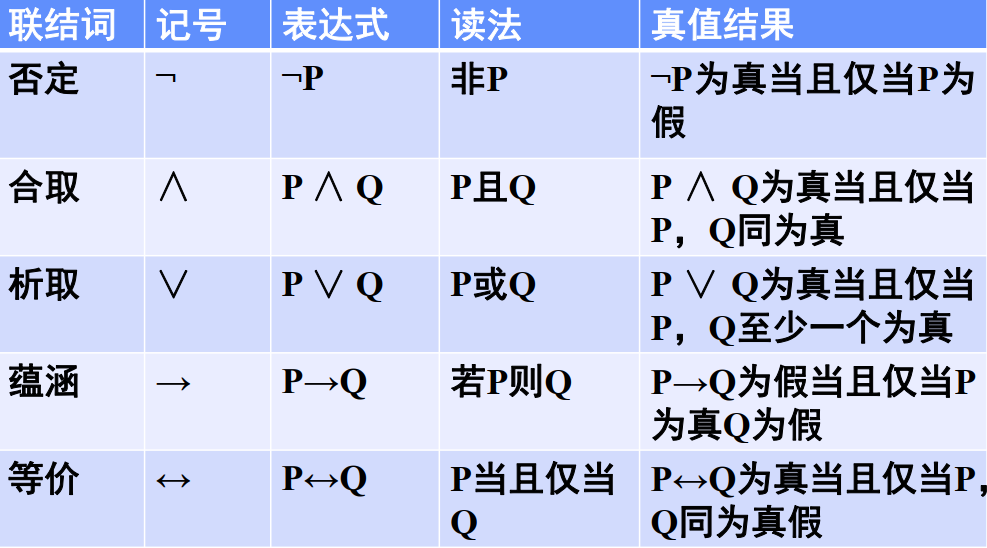一般约定：

• 运算符（联结词）结合力强弱顺序为

凡符合此顺序的，括号可省略；

• 相同的运算符，从左到右次序计算时，括号可省去；

• 最外层括号可省.

### 1.2 公式的解释与真值表

#### 1.2.1 命题公式

定义1.7

一个特定的命题是一个常值命题（constant proposition），它不是具有值“ $\mathrm{T}$$\mathrm T$ ”（“$0$$0$”）就是具有值“ $\mathrm{F}$$\mathrm F$ ”（“$0$$0$”）；

一个任意的没有赋予具体内容的原子命题是一个变量命题，常称它为命题变量（命题变元、命题变项）（proposition variable），命题变量无具体的真值，它的值域是集合 $\left\{\mathrm{T},\mathrm{F}\right\}$$\{\mathrm T,\mathrm F\}$ （或 $\{1,\ 0\}$）.

原子命题在不指派真值时称为命题变元，而复合命题由原子命题和联结词构成，可以看作是命题变元的函数，且该函数的值仍为“真”或“假”，可以称为真值函数（true value function）或命题公式（propositional formula）.

不是原子命题和联结词的任意组合都可以为命题公式. 我们用递归的方法来定义命题公式.

定义1.8 命题公式（合式公式、形式命题）

1. 命题变元本身是一个公式；

2. 如果 $P$ 是公式，则 $\mathrm{¬}P$$\lnot P$ 也是公式；

3. 如果 $P$$Q$$Q$ 是公式，则 $P\wedge Q$$P\land Q$$P\vee Q$$P\lor Q$$P\to Q$$P\to Q$$P↔Q$$P\leftrightarrow Q$ 也是公式；

4. 命题公式是仅由有限步使用规则 1 ~ 3 后产生的结果，公式常用符号 $G$$H$$H$ 等表示.

注意：

• 如果 $G$ 是含有 $n$$n$ 个命题变元 $P_1,\ P_2,\cdots,\ P_n$ 的公式，则 $G$ 通常记为 $G(P_1,\ P_2,\cdots,\ P_n)$ 或简记为 $G$.

• 原子命题变元是最简单的公式，也叫原子公式；

• 合式公式没有真值，只有对其变元进行指派后才有真值；

• 合式公式无限多.

#### 1.2.2 公式的解释与真值表

公式本身没有真值，只有在对其所有命题变元指定真值后才变成一个具有真值的命题.

定义1.9 公式的解释

$P_1,\ P_2,\cdots,\ P_n$ 是出现在公式 $G$ 中的所有命题变元，指定 $P_1,\ P_2,\cdots,\ P_n$ 一组真值，则这组真值称为 $G$ 的一个解释（explanation）或真值指派，记作 $I$$I$.

一般来说，若有 $n$$n$ 个命题变元，则应有 ${2}^{n}$$2^n$ 个不同的解释.

定义1.10 公式的真值表

公式 $G$ 在其所有可能的解释下所取真值的表，称作 $G$ 的真值表（truth）.

#### 1.2.3 一些特殊的公式

##### 1. 重言式

定义1.11

• 公式 $G$ 称为永真公式（重言式），如果在它的所有解释下都为“真”，记作 $\models G$$\vDash G$

• 公式 $G$ 称为可满足的，如果它不是永假的；

• 公式 $G$ 称为永假公式（矛盾式），如果在它的所有解释下都为“假”，有时也称为不可满足公式.

注意：

• 重言式的否定是矛盾式，矛盾式的否定是重言式，因此只需研究其中一种情形；

• 重言式的合取、析取、蕴涵、等价等都是重言式；

• 重言式中有许多非常有用的恒等式和永真蕴涵式；

• 命题的永真（永假）性是可判定的，其判定方法有真值表法和公式推演法.

##### 2. 恒等式

定义1.12

公式 $G$$H$$H$，如果在其任意解释下，其真值相同，则称 $G$$H$$H$ 的等价式（equivalent）或称 $G$ 恒等于 $H$$H$，记作 $G⇔H$$G\Leftrightarrow H$$\models G↔H$$\vDash G\leftrightarrow H$.

定理1.1

对于公式 $G$$H$$H$$G⇔H$$G\Leftrightarrow H$ 的充分必要条件是公式 $G↔H$$G\leftrightarrow H$ 是重言式.

常用逻辑恒等式：

• E1 双重否定律：

$\mathrm{¬}\mathrm{¬}P⇔P；$
• E2 $\vee$$\lor$ 的幂等律：

$P\vee P⇔P;$
• E3 $\wedge$$\land$ 的幂等律：

$P\wedge P⇔P;$
• E4 $\vee$$\lor$ 的交换律：

$P\vee Q⇔Q\vee P;$
• E5 $\wedge$$\land$ 的交换律：

$P\wedge Q⇔Q\wedge P;$
• E6 $\vee$$\lor$ 的结合律：

$\left(P\vee Q\right)\vee R⇔P\vee \left(Q\vee R\right);$
• E7 $\wedge$$\land$ 的结合律：

$\left(P\wedge Q\right)\wedge R⇔P\wedge \left(Q\wedge R\right);$
• E8 $\wedge$$\land$$\vee$$\lor$ 上的分配律：

$P\wedge \left(Q\vee R\right)⇔\left(P\wedge Q\right)\vee \left(P\wedge R\right);$
• E9 $\vee$$\lor$$\wedge$$\land$ 上的分配律：

$P\vee \left(Q\wedge R\right)⇔\left(P\vee Q\right)\wedge \left(P\vee R\right);$
• E10、E11 德·摩根定律（De Morgan's Law）:

$\begin{array}{rl}\mathrm{¬}\left(P\vee Q\right)& ⇔\mathrm{¬}P\wedge \mathrm{¬}Q,\\ \\ \mathrm{¬}\left(P\wedge Q\right)& ⇔\mathrm{¬}P\vee \mathrm{¬}Q;\end{array}$
• E12、E13 吸收律：

$\begin{array}{rl}P\vee \left(P\wedge Q\right)& ⇔P,\\ \\ P\wedge \left(P\vee Q\right)& ⇔P;\end{array}$
• E14 蕴涵等值式：

$\left(P\to Q\right)⇔\mathrm{¬}P\vee Q;$
• E15 等价等值式：

$\left(P↔Q\right)⇔\left(P\to Q\right)\wedge \left(Q\to P\right);$
• E16、E17 零律：

$\begin{array}{rl}P\vee \mathrm{T}& ⇔\mathrm{T},\\ \\ P\wedge \mathrm{F}& ⇔\mathrm{F};\end{array}$
• E18、E19 幺律：

$\begin{array}{rl}P\vee \mathrm{F}& ⇔P,\\ \\ P\wedge \mathrm{T}& ⇔P;\end{array}$
• E20 排中律：

$P\vee \mathrm{¬}P⇔\mathrm{T};$
• E21 矛盾律：

$P\wedge \mathrm{¬}P⇔\mathrm{F};$
• E22 等价否定等值式：

$\left(P↔Q\right)⇔\left(\mathrm{¬}P↔\mathrm{¬}Q\right);$
• E23 归谬律：

$\left(\left(P\to Q\right)\wedge \left(P\to \mathrm{¬}Q\right)\right)⇔\mathrm{¬}P;$
• E24 逆反律（假言异位）：

$\left(P\to Q\right)⇔\mathrm{¬}Q\to \mathrm{¬}P.$
##### 3. 永真蕴涵式

定义1.13

$A\to B$$A\to B$ 是一永真式，那么称其为永真蕴涵式，记为 $A⇒B$$A\Rightarrow B$，读作“$A$ 永真蕴涵 $B$$B$”.

常用永真蕴涵式：

• I1 同一律

$P⇒P;$
• I2

$P⇒P\vee Q;$
• I3

$P\wedge Q⇒P;$
• I4

$P\wedge \left(P\to Q\right)⇒Q;$
• I5

$\left(P\to Q\right)\wedge \mathrm{¬}Q⇒\mathrm{¬}P;$
• I6

$\mathrm{¬}P\wedge \left(P\vee Q\right)⇒Q;$
• I7

$\left(P\to Q\right)\wedge \left(Q\to R\right)⇒\left(P\to R\right);$
• I8

$\left(P\to Q\right)⇒\left(\left(Q\to R\right)\to \left(P\to R\right)\right);$
• I9

$\left(\left(P\to Q\right)\wedge \left(R\to S\right)\right)⇒\left(P\wedge R\to Q\wedge S\right).$
##### 4. 恒等式和永真蕴涵式的两个性质
• 逻辑恒等（“$⇔$$\Leftrightarrow$”）和永真蕴涵（“$⇒$$\Rightarrow$”）都是可传递的；

• $A⇒B$$A\Rightarrow B$$B⇒C$$B\Rightarrow C$，则 $A⇒B\wedge C$$A\Rightarrow B\land C$.

#### 1.2.4 代入规则和替换规则

定理1.2 代入定理

$G\left({P}_{1},\cdots ,{P}_{n}\right)$$G(P_1,\cdots,P_n)$ 是一个命题公式，其中 ${P}_{1},\cdots ,{P}_{n}$$P_1,\cdots,P_n$ 是命题变元，${G}_{1}\left({P}_{1},\cdots ,{P}_{n}\right)$$G_1(P_1,\cdots,P_n)$$\cdots$$\cdots$${G}_{n}\left({P}_{1},\cdots ,{P}_{n}\right)$$G_n(P_1,\cdots,P_n)$ 为任意的命题公式，此时若 $G$ 是永真公式或永假公式，则用 ${G}_{1}$$G_1$ 取代 ${P}_{1}$$P_1$${G}_{n}$$G_n$ 取代 ${P}_{n}$$P_n$ 后得到的新命题公式

$G\left({G}_{1},\cdots ,{G}_{n}\right)⇔{G}^{\prime }\left({P}_{1},\cdots ,{P}_{n}\right)$

也是一个永真公式或永假公式.

定理1.3 替换定理

${G}_{1}$$G_1$$G$ 的子公式，${H}_{1}$$H_1$ 是任意的命题公式，在 $G$ 中凡出现 ${G}_{1}$$G_1$ 处都以 ${H}_{1}$$H_1$ 替换后得到新的命题公式 $H$$H$. 若 ${G}_{1}⇔{H}_{1}$$G_1\Leftrightarrow H_1$，则 $G⇔H$$G\Leftrightarrow H$.

#### 1.2.5 对偶原理

定义1.4 对偶公式

设公式 $A$ 中仅有联结词 $\wedge$$\land$$\vee$$\lor$$\mathrm{¬}$$\lnot$. 在 $A$ 中将 $\wedge$$\land$$\vee$$\lor$$\mathrm{T}$$\mathrm{T}$$\mathrm{F}$$\mathrm{F}$ 分别换以 $\vee$$\lor$$\wedge$$\land$$\mathrm{F}$$\mathrm{F}$$\mathrm{T}$$\mathrm{T}$ 得到公式 ${A}^{\ast }$$A^*$，则 ${A}^{\ast }$$A^*$ 称为 $A$ 的对偶公式，$A$${A}^{\ast }$$A^*$ 互为对偶.

定理1.4

$A$${A}^{\ast }$$A^*$ 是对偶式，${P}_{1},{P}_{2},\cdots ,{P}_{n}$$P_1,P_2,\cdots,P_n$ 是出现于 $A$${A}^{\ast }$$A^*$ 中所有命题变元，于是

$\begin{array}{}\text{(1)}& \mathrm{¬}A\left({P}_{1},{P}_{2},\cdots ,{P}_{n}\right)={A}^{\ast }\left(\mathrm{¬}{P}_{1},\mathrm{¬}{P}_{2},\cdots ,\mathrm{¬}{P}_{n}\right).\end{array}$

定理1.5 对偶原理

$A⇔B$$A\Leftrightarrow B$，且 $A$$B$$B$ 为命题变元 ${P}_{1},{P}_{2},\cdots ,{P}_{n}$$P_1,P_2,\cdots,P_n$ 及联结词 $\wedge ,\vee ,\mathrm{¬}$$\land,\lor,\lnot$ 构成的公式，则 ${A}^{\ast }⇔{B}^{\ast }$$A^*\Leftrightarrow B^*$.

定理1.6

$A⇒B$$A\Rightarrow B$，且 $A$$B$$B$ 为命题变元 ${P}_{1},{P}_{2},\cdots ,{P}_{n}$$P_1,P_2,\cdots,P_n$ 及联结词 $\wedge ,\vee ,\mathrm{¬}$$\land,\lor,\lnot$ 构成的公式，则 ${B}^{\ast }⇔{A}^{\ast }$$B^*\Leftrightarrow A^*$.

### 1.3 联结词的完备集

#### 1.3.1 联结词的扩充

首先考虑一元运算.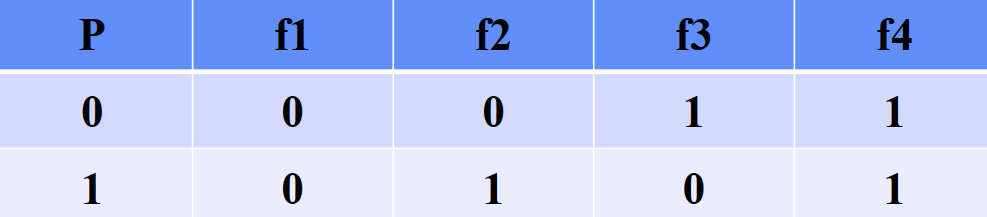最多只能定义 4 种运算，但除了否定之外，永假、永真、恒等作为运算意义不大，所以一般不再定义其它一元运算.

考虑两变元在一个二元运算作用下可能的真值表.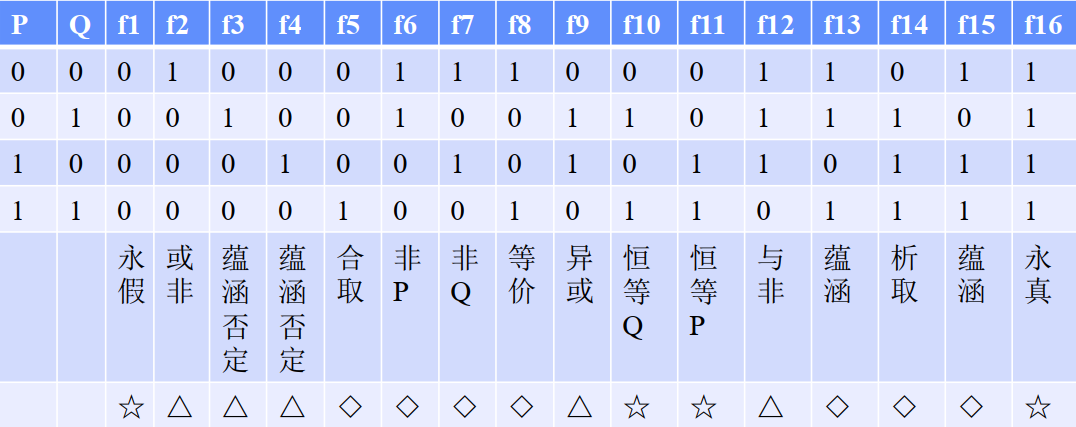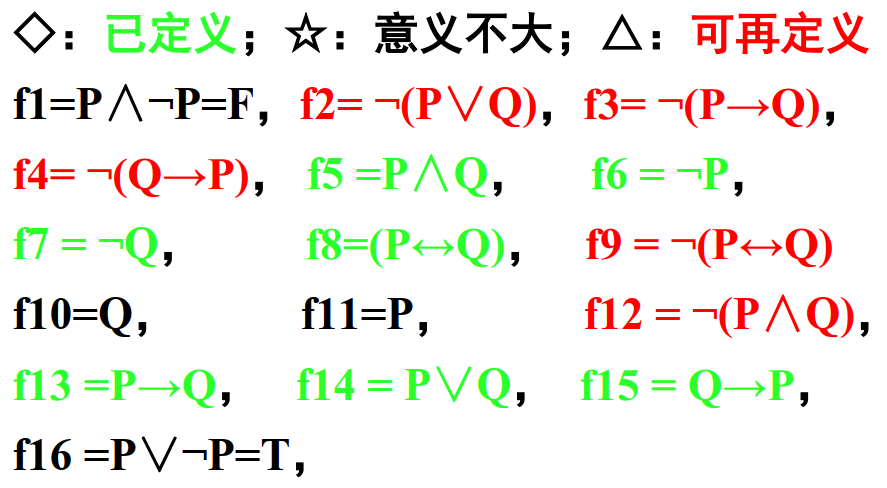为了叙述方便，引进下面四个联结词.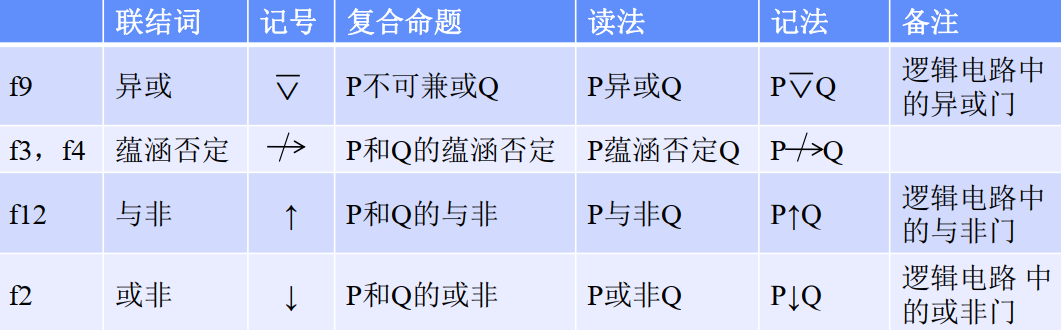$\begin{array}{rl}P\stackrel{―}{\vee }Q& ⇔\mathrm{¬}\left(P↔Q\right)\\ P↛Q& ⇔\mathrm{¬}\left(P\to Q\right)\\ P↑Q& ⇔\mathrm{¬}\left(P\wedge Q\right)\\ P↓Q& ⇔\mathrm{¬}\left(P\vee Q\right)\end{array}$

这九个联结词（否定、合取、析取、蕴涵、等价、异或、蕴含否定、与非、或非）构成命题演算中的所有联结词.

#### 1.3.2 与非、或非、异或的性质

与非的性质：

• $P↑Q⇔Q↑P$$P\uparrow Q\Leftrightarrow Q\uparrow P$

• $P↑P⇔\mathrm{¬}P$$P\uparrow P\Leftrightarrow\lnot P$

• $\left(P↑Q\right)↑\left(P↑Q\right)⇔P\wedge Q$$(P\uparrow Q)\uparrow(P\uparrow Q)\Leftrightarrow P\land Q$

• $\left(P↑P\right)↑\left(Q↑Q\right)⇔P\vee Q$$(P\uparrow P)\uparrow(Q\uparrow Q)\Leftrightarrow P\lor Q$.

或非的性质：

• $P↓Q⇔Q↓P$$P\downarrow Q\Leftrightarrow Q\downarrow P$

• $P↓P⇔\mathrm{¬}P$$P\downarrow P\Leftrightarrow\lnot P$

• $\left(P↓Q\right)↓\left(P↓Q\right)⇔P\vee Q$$(P\downarrow Q)\downarrow(P\downarrow Q)\Leftrightarrow P\lor Q$

• $\left(P↓P\right)↓\left(Q↓Q\right)⇔P\wedge Q$$(P\downarrow P)\downarrow(Q\downarrow Q)\Leftrightarrow P\land Q$.

异或的性质：

• $P\stackrel{―}{\vee }Q⇔Q\stackrel{―}{\vee }P$$P\overline{\lor}Q\Leftrightarrow Q\overline{\lor} P$

• $P\stackrel{―}{\vee }\left(Q\stackrel{―}{\vee }R\right)⇔\left(P\stackrel{―}{\vee }Q\right)\stackrel{―}{\vee }R$$P\overline{\lor}(Q\overline{\lor}R)\Leftrightarrow(P\overline{\lor}Q)\overline{\lor}R$

• $P\stackrel{―}{\vee }P⇔\mathrm{F}$$P\overline{\lor}P\Leftrightarrow\mathrm{F}$

• $\mathrm{F}\stackrel{―}{\vee }P⇔P$$\mathrm{F}\overline{\lor}P\Leftrightarrow P$

• $\mathrm{T}\stackrel{―}{\vee }P⇔\mathrm{¬}P$$\mathrm{T}\overline{\lor}P\Leftrightarrow \lnot P$.

#### 1.3.3 全功能联结词

定义1.15

$S$$S$ 的联结词的集合.

1. $S$$S$ 中的联结词表示的公式可以等价地表示任何命题公式，则称 $S$$S$ 是一个联结词完备集（或全功能集合）（adequate set of connectives）；

2. $S$$S$ 是一个联结词的完备集，且 $S$$S$ 中无冗余的联结词（即集合中不存在可以被其中的其它联结词所定义的联结词），则称 $S$$S$ 为极小联结词完备集.

• $\left\{\mathrm{¬},\wedge \right\}$$\{\lnot,\land\}$$\left\{\mathrm{¬},\vee \right\}$$\{\lnot,\lor\}$$\left\{\mathrm{¬},\to \right\}$$\{\lnot,\to\}$$\left\{\mathrm{¬},↛\right\}$$\{\lnot,\nrightarrow\}$$\left\{↑\right\}$$\{\uparrow\}$$\left\{↓\right\}$$\{\downarrow\}$ 都是极小完备集；

• $\left\{\wedge ,\vee ,\to ,↔\right\}$$\{\land,\lor,\to,\leftrightarrow\}$ 及其子集不是完备集；

• 实际应用中经常采取的联结词集合为 $\left\{\mathrm{¬},\wedge ,\vee \right\}$$\{\lnot,\land,\lor\}$.

### 1.4 范式

#### 1.4.1 范式

同一个命题有不同的表达形式，这样给命题演算带来了一定的困难. 为了使命题公式规范化，引入范式的概念.

定义1.16

• 命题变元或命题变元的否定称为文字；

• 有限个文字的析取式称为简单析取式（基本和），有限个文字的合取式称为简单合取式（基本积）；

• 由有限个简单合取式构成的析取式称为析取范式（disjunctive normal form），由有限个简单析取式构成的合取式称为合取范式（conjunctive normal form）.

性质：

• 一个文字既是一个析取范式又是一个合取范式；

• 一个析取范式为矛盾式当且仅当它的每个简单合取式是矛盾式；

• 一个合取范式为重言式当且仅当它的每个简单析取式是重言式.

定理1.7

任一命题公式都存在着与之等值的析取范式和合取范式.

证明：对于任一公式，可用下面的方法构造出与之等值的范式：

1. 利用等价公式

使公式中仅含联结词 $\mathrm{¬}$$\lnot$$\wedge$$\land$$\vee$$\lor$

2. 利用德·摩根定律

和双重否定律

$\mathrm{¬}\mathrm{¬}A=A$

将否定符 $\mathrm{¬}$$\lnot$ 移到命题变元前，并去掉多余的否定符；

3. 利用分配律

将公式化成析取范式或合取范式，所得即与原公式等价.

#### 1.4.2 主析取范式和主合取范式

范式虽然为命题公式提供了一种统一的表达形式，但这种表达形式却不是唯一的，这种不唯一的表达形式给研究问题带来了不便，因此有必要引进更为标准的范式.

定义1.17

• 包含 $A$ 中所有命题变元或其否定一次仅一次的简单合取式称为极小项；

• 包含 $A$ 中所有命题变元或其否定一次仅一次的简单析取式称为极大项；

• 由有限个极小项组成的析取范式称为主析取范式；

• 由有限个极大项组成的合取范式称为主合取范式.

##### 1. 极小项和极大项的性质

一般来说，对于 $n$$n$ 个命题变元，应有 ${2}^{n}$$2^n$ 个不同的极小项和 ${2}^{n}$$2^n$ 个不同的极大项.

性质：

• 没有两个不同的极小项是等价的，且每个极小项只有一组真值指派使得该极小项的真值为真，因此可给极小项编码，使极小项为 $\mathrm{T}$$\mathrm{T}$ 的那组真值指派为对应的极小项编码；

• 没有两个不同的极大项是等价的，且每个极大项只有一组真值指派使得该极大项的真值为假，因此可给极小项编码，使极小项为 $\mathrm{F}$$\mathrm{F}$ 的那组真值指派为对应的极大项编码.

• 任意两极小项的合取必假，任意两极大项的析取必真，

极大项的否定是极小项，极小项的否定是极大项，

• 所有极小项的析取为永真公式，所有极大项的合取为永假公式，即

##### 2. 主析取范式和主合取范式的存在性和唯一性

定理1.8

任何命题公式的主析取范式和主合取范式存在且唯一，即任何命题公式有且仅有一个与之等价的主析取范式和主合取范式.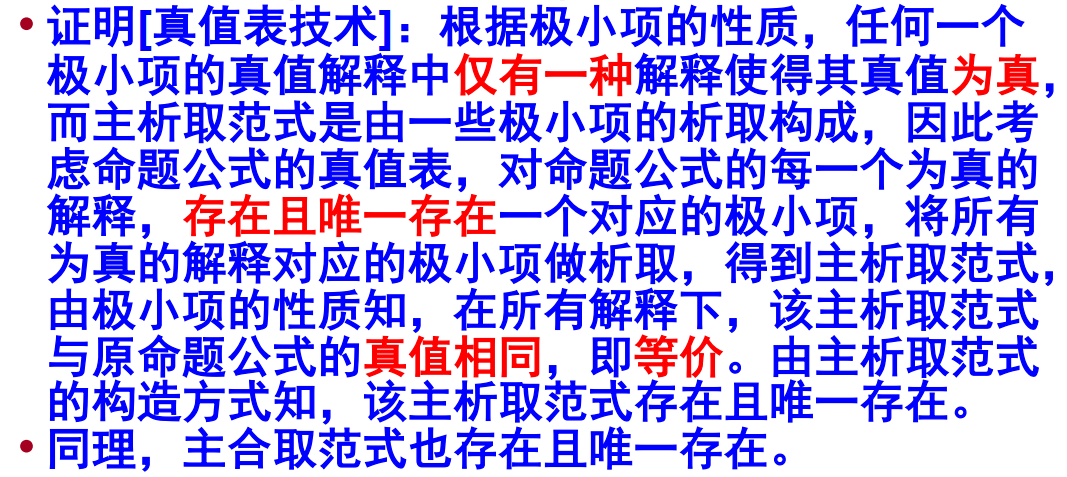求主析取范式和主合取范式的方法：

• 真值表技术：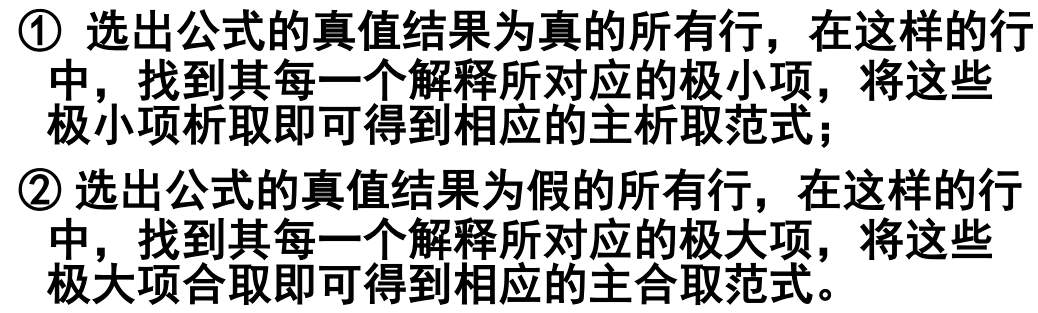• 公式转换法：在公式中的命题变元较少时，利用真值表技术更为简单；当命题变元较多时，一般采用公式转换法.

##### 3. 主析取范式和主合取范式之间的转换

(1) 已知公式 $G$ 的主析取范式，求公式 $G$ 的主合取范式：

• $\mathrm{¬}G$$\lnot G$ 的主析取范式，即 $G$ 的主析取范式中没有出现过的极小项的析取；

• $G⇔\mathrm{¬}\left(\mathrm{¬}G\right)$$G\Leftrightarrow\lnot(\lnot G)$ 即是 $G$ 的主合取范式.

即，若

$G=\underset{i}{\overset{k}{\bigvee }}{m}_{{j}_{i}}$

$G$ 的主析取范式，则

$\mathrm{¬}G=\underset{i=1}{\overset{{2}^{n}-k}{\bigvee }}{m}_{{j}_{i}^{\prime }}$

$\mathrm{¬}G$$\lnot G$ 的主析取范式，其中 ${m}_{{j}_{i}^{\prime }}、\left(i=1,2,\cdots ,{2}^{n}-k\right)$$m_{j_i'}、 (i=1,2,\cdots,2^n-k)$$m_i\ (i=0,1,\cdots,2^n-1)$ 中去掉 $m_{j_i}\ (i=1,\cdots,k)$ 后剩下的极小项，则

$G⇔\mathrm{¬}\left(\mathrm{¬}G\right)⇔\mathrm{¬}\left(\underset{i=1}{\overset{{2}^{n}-k}{\bigvee }}{m}_{{j}_{i}^{\prime }}\right)⇔\underset{i=1}{\overset{{2}^{n}-k}{\bigwedge }}\mathrm{¬}{m}_{{j}_{i}^{\prime }}⇔\underset{i=1}{\overset{{2}^{n}-k}{\bigwedge }}{M}_{{j}_{i}^{\prime }}.$

(2) 已知公式 $G$ 的主合取范式，求公式 $G$ 的主析取范式：

• $\mathrm{¬}G$$\lnot G$ 的主合取范式，即 $G$ 的主合取范式中没有出现过的极大项的合取；

• $G⇔\mathrm{¬}\left(\mathrm{¬}G\right)$$G\Leftrightarrow\lnot(\lnot G)$ 即是 $G$ 的主析取范式.

即，若

$G=\underset{i}{\overset{k}{\bigwedge }}{M}_{{j}_{i}}$

$G$ 的主合取范式，则

$\mathrm{¬}G=\underset{i=1}{\overset{{2}^{n}-k}{\bigwedge }}{M}_{{j}_{i}^{\prime }}$

$\mathrm{¬}G$$\lnot G$ 的主合取范式，其中 ${M}_{{j}_{i}^{\prime }}、\left(i=1,2,\cdots ,{2}^{n}-k\right)$